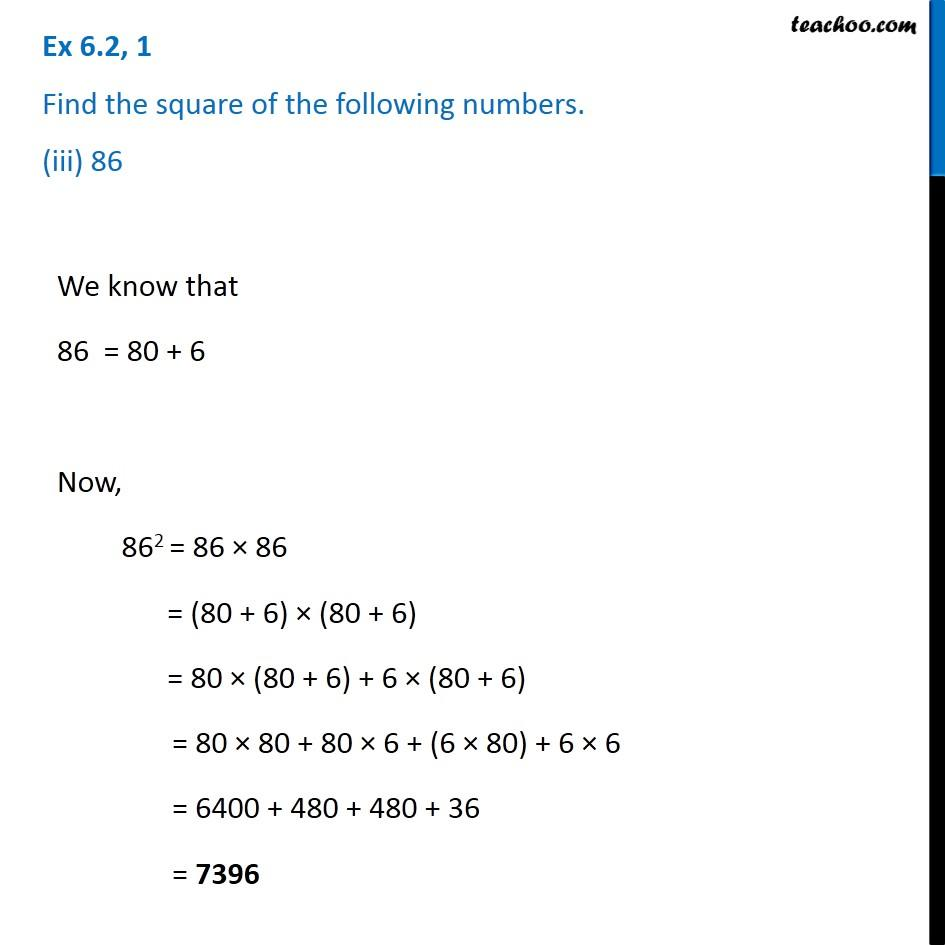Ex 6.2

Chapter 6 Class 8 Squares and Square Roots
Serial order wiseGet live Maths 1-on-1 Classs - Class 6 to 12

### Transcript

Ex 6.2, 1 Find the square of the following numbers. (iii) 86We know that 86 = 80 + 6 Now, 862 = 86 × 86 = (80 + 6) × (80 + 6) = 80 × (80 + 6) + 6 × (80 + 6) = 80 × 80 + 80 × 6 + (6 × 80) + 6 × 6 = 6400 + 480 + 480 + 36 = 7396